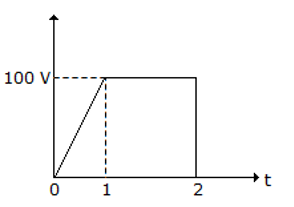# UPRVUNL’21 EE: Weekly Practice Quiz-01

Each question carries 1 mark.
Negative marking: 1/4 mark
Total Questions: 12
Time: 12 min.

Q1. In a transformer, Low voltage windings are placed nearer to the core in the case of concentric windings because
(a) it reduces hysteresis loss
(b) it reduces eddy current loss
(c) it reduces insulation requirement
(d) it reduces leakage fluxes

Q2. Reactance relay is used for protection in:
(a) Long transmission lines
(b) Short transmission lines
(c) Medium transmission lines
(d) Both long transmission lines and short transmission lines

Q3. The rms value of wave in figure isQ4. The iron losses are neglected during short circuit test of a transformer because:
(a) The flux produced is very small as compared to the normal flux
(b) Copper losses include iron losses
(c) The iron core is fully saturated
(d) The supply frequency influences copper losses

Q5. For minimum weight of the transformer, the iron weight should be……. the weight of the copper.
(a) More than
(b) Equal to
(c) Less than
(d) No relation

Q6. What KVA rating is required for a transformer that must handle a maximum load current of 8 A with a secondary voltage of 2 KV?
(a) O.25 KVA
(b) 4 KVA
(c) 8 KVA
(d) 16 KVA

Q7. The speed of a dc series motor decreases if the flux in the field winding
(a) Remains constant
(b) Increases
(c) Decreases
(d) None of these

Q8. When is the mechanical power developed by a dc motor maximum?
(E_b=back emf,V=applied voltage)
(a) E_b=V
(b) E_b=0
(c) E_b=V/2
(d) None of the above

Q9. If, in a DC machine, the ratio of constant losses to armature resistance is 2, then at maximum efficiency, the magnitude of current flowing would be:
(a) 2 A
(b) 4 A
(c) 6.667 A
(d) 1.414 A

Q10. Which of the following statements is/are correct statements?
(a) For ideal transformer efficiency is 100%
(b) For ideal transformer voltage regulation is 100%
(c) both a and b
(d) none of the above

Q11. A current of 3A through a coil sets flux linkage of 15 Wb-turn. The inductance of the coil is?
(a) 1 H
(b) 3 H
(c) 5 H
(d) 15 H

Q12. Which of the following is bilateral element?
(a) Constant voltage source
(b) Constant current source
(C) Capacitance
(d) All of the above

SOLUTIONS
S1. Ans.(c)
Sol. In concentric arrangement of placing of HV and LV winding, less insulation is required when LV winding is placed near the core which is at ground potential. The HV winding is placed around the LV.
If high voltage winding is placed near the core, the more insulation will be required and the more insulation led to increase in the size and cost of the transformer.

S2. Ans.(b)
Sol. Relay used for different configuration of transmission lines……
Short transmission line: reactance relay
Medium transmission line: impedance relay
Long transmission line: mho relay

S3. Ans.(b)
Sol.

Here, f(t)={█(100t; 0<t<1@ 100 ; 1<t<2 )┤

RMS= √(1/2 {∫_0^1▒〖(100t)^2 dt+∫_1^2▒〖(100)^2 dt〗〗} )≃80 V

S4. Ans.(a)
Sol. The iron losses are neglected during short circuit test of a transformer because voltage applied across the HV (High Voltage) side is a small fraction of the rated voltage and so its flux.

S5. Ans.(b)
Sol. For minimum weight of transformer: weight of iron=weight of copper
i.e., G_i=G_c

S6. Ans.(d)
Sol. KVA rating=V_s I_s=2×8=16 KVA

S7. Ans.(b)
Sol. N α 1/ ϕ
So, the speed of a dc series motor decreases if the flux in the field winding increases.

S8. Ans.(c)
Sol. In case of DC motor, to develop maximum mechanical power, E_b=V/2.

S9. Ans.(d)
Sol. For maximum efficiency, I_a^2 R_a=P_i
∴I_a=√(P_i/R_a )=√2=1.414 A

S10. Ans.(c)
Sol. For any ideal device efficiency should be 100% and its voltage regulation should be zero means there should not be any internal losses are there and no change in secondary voltage due to load.

S11. Ans.(c)
Sol. inductance of coil=L=Nϕ/I=15/3=5 H

S12. Ans.(c)
Sol. Bilateral elements are defined as the elements through which magnitude of current is independent of polarity of supply voltage.
e.g., of bilateral elements: resistors, inductors, capacitors.

×Join India's largest learning destination

What You Will get ?

•Job Alerts
•Daily Quizzes
•Subject-Wise Quizzes
•Current Affairs
•Previous year question papers
•Doubt Solving session

ORJoin India's largest learning destination

What You Will get ?

•Job Alerts
•Daily Quizzes
•Subject-Wise Quizzes
•Current Affairs
•Previous year question papers
•Doubt Solving session

ORJoin India's largest learning destination

What You Will get ?

•Job Alerts
•Daily Quizzes
•Subject-Wise Quizzes
•Current Affairs
•Previous year question papers
•Doubt Solving session

Enter the email address associated with your account, and we'll email you an OTP to verify it's you.Join India's largest learning destination

What You Will get ?

•Job Alerts
•Daily Quizzes
•Subject-Wise Quizzes
•Current Affairs
•Previous year question papers
•Doubt Solving session

Enter OTP

Please enter the OTP sent to
/6

Did not recive OTP?

Resend in 60sJoin India's largest learning destination

What You Will get ?

•Job Alerts
•Daily Quizzes
•Subject-Wise Quizzes
•Current Affairs
•Previous year question papers
•Doubt Solving sessionJoin India's largest learning destination

What You Will get ?

•Job Alerts
•Daily Quizzes
•Subject-Wise Quizzes
•Current Affairs
•Previous year question papers
•Doubt Solving session

Almost there

+91Join India's largest learning destination

What You Will get ?

•Job Alerts
•Daily Quizzes
•Subject-Wise Quizzes
•Current Affairs
•Previous year question papers
•Doubt Solving session

Enter OTP

Please enter the OTP sent to Edit Number

Did not recive OTP?

Resend 60

By skipping this step you will not recieve any free content avalaible on adda247, also you will miss onto notification and job alerts

Are you sure you want to skip this step?

By skipping this step you will not recieve any free content avalaible on adda247, also you will miss onto notification and job alerts

Are you sure you want to skip this step?